# Introduction to splines2

#### 2018-06-14

The package splines2 is designed to be a supplementary package on splines. It provides functions constructing a variety of spline bases that are not available from the package splines shipped with R. Most functions have a very similar user interface with the function bs in package splines. Currently, splines2 provides function constructing B-splines, integral of B-splines, monotone splines (M-splines) and its integral (I-splines), convex splines (C-splines), and their derivatives. Compared with package splines, splines2 allows piecewise constant basis for B-splines. Also, it provides a more user-friendly function interface, more consistent handling on NA’s for spline derivatives.

In this vignette, we introduce the basic usage of the functions provided by examples. The details of function syntax are available in the package manual and thus will be not discussed.

An outline of the remainder of the vignette is as follows: We first introduce the functions constructing the monotone splines (M-splines), its integral (I-splines), and convex splines (C-splines). The deriv methods for derivatives is demonstrated at the same time. After then, toy examples for integral and derivative of B-splines and B-splines, M-splines allowing piecewise constant are given. Last but not the least, handy methods of S3 generic function predict for objects produced by splines2 are demonstrated for the evaluation of the same spline basis at new values.

## M-splines using mSpline

M-splines (Ramsay 1988) can be considered as a normalized version of B-splines with unit integral within boundary knots. An example given by Ramsay (1988) was a quadratic M-splines with three internal knots placed at 0.3, 0.5, and 0.6. The boundary knots by default are the range of the data x, thus 0 and 1 in this example.

library(splines2)
knots <- c(0.3, 0.5, 0.6)
x <- seq(0, 1, 0.01)
msOut <- mSpline(x, knots = knots, degree = 2, intercept = TRUE)
library(graphics) # attach graphics (just in case) for plots
par(mar = c(2.5, 2.5, 0, 0), mgp = c(1.5, 0.5, 0))
matplot(x, msOut, type = "l", ylab = "y")
abline(v = knots, lty = 2, col = "gray") # mark internal knots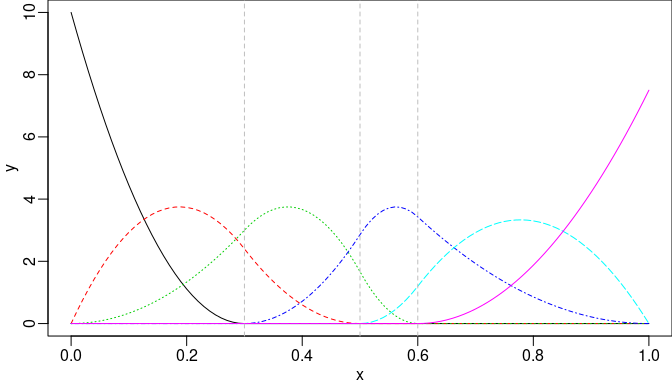Quadratic M-splines with three internal knots.

The derivative of given order of M-splines can be obtained by specifying a positive integer to argument dervis of mSpline. Also, for an existing mSpline object generated from function mSpline, the deriv method can be used conveniently. For example, the first derivative of the M-splines given in last example can be obtained equivalently as follows:

dmsOut1 <- mSpline(x, knots = knots, degree = 2, intercept = TRUE, derivs = 1)
dmsOut2 <- deriv(msOut)
stopifnot(all.equal(dmsOut1, dmsOut2, check.attributes = FALSE))

## I-splines using iSpline

I-splines (Ramsay 1988) are simply the integral of M-splines and thus monotonically non-decreasing with unit maximum value. A monotonically non-decreasing (non-increasing) function can be fitted by a linear combination of I-spline bases with non-negative (non-positive) coefficients, plus a constant function (where the coefficient of the constant function is unconstrained).

The example given by Ramsay (1988) was the I-splines corresponding to that quadratic M-splines with three internal knots placed at 0.3, 0.5, and 0.6. Note that the degree of I-splines is defined from the associated M-splines instead of their own polynomial degree.

isOut <- iSpline(x, knots = knots, degree = 2, intercept = TRUE)
par(mar = c(2.5, 2.5, 0, 0), mgp = c(1.5, 0.5, 0))
matplot(x, isOut, type = "l", ylab = "y")
abline(h = 1, v = knots, lty = 2, col = "gray")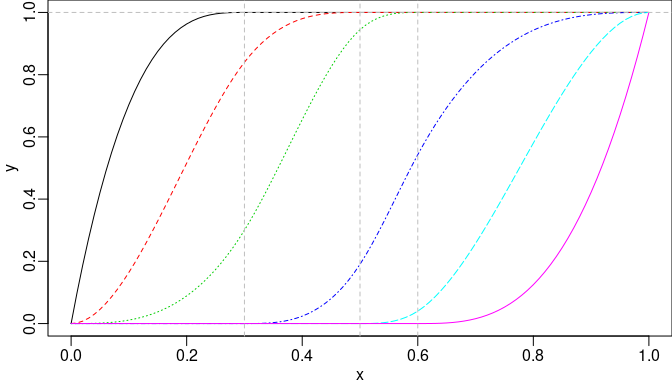I-splines of degree two with three internal knots.

The corresponding M-spline basis matrix can be obtained easily by the deriv method, which internally exacts the attribute named msMat in the object returned by function iSpline. In other words, if we need both M-spline bases and their integral splines in model fitting, iSpline and its deriv method should be used, while an extra function call of mSpline should be avoided for a better performance.

stopifnot(all.equal(msOut, deriv(isOut)))

## C-splines using cSpline

Convex splines (Meyer 2008) called C-splines are a scaled version of I-splines’ integral with unit maximum value. Meyer (2008) applied C-splines to shape-restricted regression analysis. The monotone property of I-spines ensures the convexity of C-splines. A convex regression function can be estimated using linear combinations of the C-spline bases with non-negative coefficients, plus an unrestricted linear combination of the constant function and the identity function $$g(x)=x$$. If the underlying regression function is both increasing and convex, the coefficient on the identity function is restricted to be nonnegative as well.

Function cSpline provides argument scale specifying whether scaling on C-spline bases is required. If scale = TRUE (by default), each C-spline basis is scaled to have unit height at right boundary knot. For its first (second) derivative, the deriv method can be used, which internally exacts the corresponding I-spline (M-spline) bases shipped in attributes isMat (msMat) scaled to the same extent. The derivatives of higher order can be obtained by specifying argument derivs in the deriv method.

csOut1 <- cSpline(x, knots = knots, degree = 2, intercept = TRUE)
par(mar = c(2.5, 2.5, 0, 0), mgp = c(1.5, 0.5, 0))
matplot(x, csOut1, type = "l", ylab = "y")
abline(h = 1, v = knots, lty = 2, col = "gray")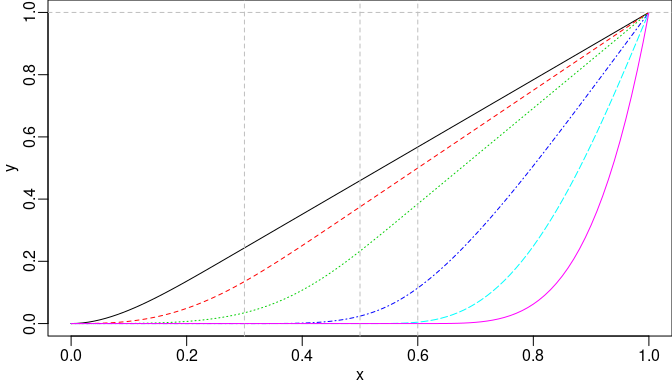C-splines of degree two with three internal knots.

If scale = FALSE, the actual integral of I-spline basis will be returned. Similarly, the corresponding deriv method is provided. For derivatives of order greater than one, the nested call of deriv is supported. However, argument derivs can be specified if possible for a better performance. For example, the first and second derivatives can be obtained by the following equivalent approaches, respectively.

csOut2 <- cSpline(x, knots = knots, degree = 2, intercept = TRUE, scale = FALSE)
stopifnot(all.equal(isOut, deriv(csOut2), check.attributes = FALSE))
stopifnot(all.equal(msOut, deriv(csOut2, 2), deriv(deriv(csOut2)),
check.attributes = FALSE))

## Integral and derivative of B-splines using ibs and dbs

A close-form recursive formulas of B-spline integral and derivative given by De Boor (1978) are implemented. Two toy example are given as follows:

ibsOut <- ibs(x, knots = knots, degree = 1, intercept = TRUE)
par(mar = c(2.5, 2.5, 0, 0), mgp = c(1.5, 0.5, 0), mfrow = c(1, 2))
matplot(x, deriv(ibsOut), type = "l", ylab = "y")
abline(v = knots, h = 1, lty = 2, col = "gray")
matplot(x, ibsOut, type = "l", ylab = "y")
abline(v = knots, h = c(0.15, 0.2, 0.25), lty = 2, col = "gray")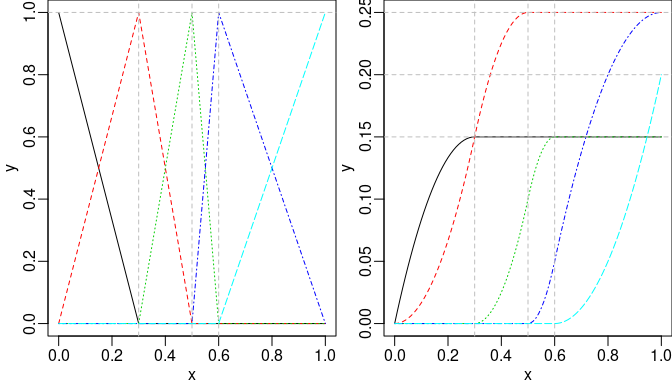Piecewise linear B-splines (left) and their integrals (right).

dbsOut <- dbs(x, knots = knots, intercept = TRUE)
bsOut <- bSpline(x, knots = knots, intercept = TRUE)
par(mar = c(2.5, 2.5, 0, 0), mgp = c(1.5, 0.5, 0), mfrow = c(1, 2))
matplot(x, bsOut, type = "l", ylab = "y")
abline(v = knots, lty = 2, col = "gray")
matplot(x, dbsOut, type = "l", ylab = "y")
abline(v = knots, lty = 2, col = "gray")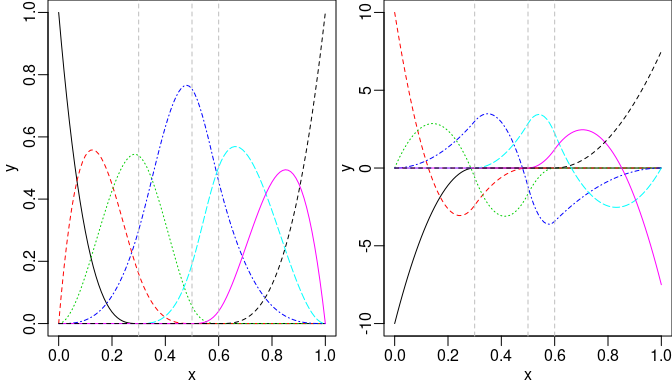Cubic B-splines (left) and their first derivative (right).

## B-splines using bSpline

Function bSpline provides B-spline bases and allows degree = 0 for piecewise constant bases, which is one simple but handy extension to function bs in package splines. (For positive degree, bSpline internally call bs to do the hard work.) Step function or piecewise constant bases (close on the left and open on the right) are often used in practice for a reasonable approximation without any assumption on the form of target function. One simple example of B-splines and M-splines of degree zero is given as follows:

bsOut0 <- bSpline(x, knots = knots, degree = 0, intercept = TRUE)
msOut0 <- mSpline(x, knots = knots, degree = 0, intercept = TRUE)
par(mar = c(2.5, 2.5, 0, 0), mgp = c(1.5, 0.5, 0), mfrow = c(1, 2))
matplot(x, bsOut0, type = "l", ylab = "y")
abline(v = knots, lty = 2, col = "gray")
matplot(x, msOut0, type = "l", ylab = "y")
abline(v = knots, lty = 2, col = "gray")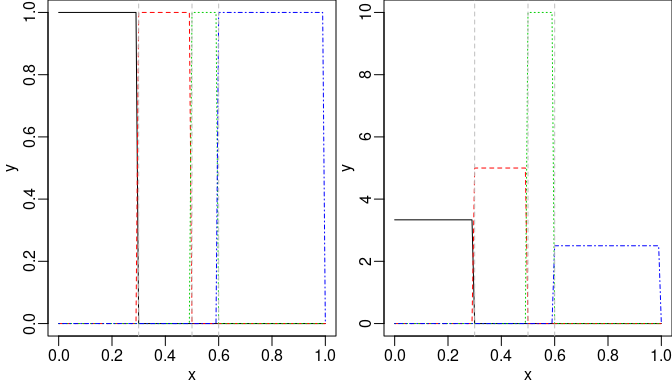B-splines (left) and M-splines (right) of degree zero

## Evaluation on New Values by predict

The methods for splines2 objects dispatched by generic function predict are useful if we want to evaluate the spline object at possibly new $$x$$ values. For instance, if we want to evaluate the value of I-splines object in previous example at 0.275, 0.525, and 0.8, respectively, all we need is

predict(isOut, c(0.275, 0.525, 0.8))
##              1         2         3         4        5     6
## [1,] 0.9994213 0.7730556 0.2310764 0.0000000 0.000000 0.000
## [2,] 1.0000000 1.0000000 0.9765625 0.2696429 0.000625 0.000
## [3,] 1.0000000 1.0000000 1.0000000 0.9428571 0.580000 0.125

Technically speaking, the methods take all information needed, such as knots, degree, intercept, etc., from attributes of the original splines2 objects and call the corresponding function automatically for those new $$x$$ values. Therefore, the predict methods will not be applicable if those attributes are somehow lost after certain operation.

## Reference

De Boor, Carl. 1978. A Practical Guide to Splines. Vol. 27. New York: springer-verlag. https://doi.org/10.1002/zamm.19800600129.

Meyer, Mary C. 2008. “Inference Using Shape-Restricted Regression Splines.” The Annals of Applied Statistics. JSTOR, 1013–33. https://doi.org/10.1214/08-AOAS167.

Ramsay, J O. 1988. “Monotone Regression Splines in Action.” Statistical Science. JSTOR, 425–41. https://doi.org/10.1214/ss/1177012761.